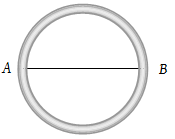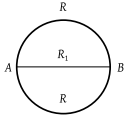The resistance of a wire is 10–6 Ω per metre. It is bend in the form of a circle of diameter 2 m. A wire of the same material is connected across its diameter. The total resistance across its diameter AB will be(1) $\frac{4}{3}\pi ×{10}^{-6}\Omega$

(2) $\frac{2}{3}\pi ×{10}^{-6}\Omega$

(3) $0.88×{10}^{-6}\Omega$

(4) $14\pi ×{10}^{-6}\Omega$

(3) Resistance across $AB=\frac{1}{R\text{'}}=\frac{1}{R}+\frac{1}{R}+\frac{1}{{R}_{1}}$${R}_{1}=2×{10}^{-6}\Omega$

and $R=\pi ×1×{10}^{-6}\Omega$

On solving,

$R\text{'}=0.88×{10}^{-6}\Omega$

Difficulty Level:

• 9%
• 37%
• 52%
• 5%